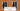# Longest Common Prefix - Leet Code Solution

September 13, 2019## Problem Statement

Write a function to find the longest common prefix string amongst an array of strings.

If there is no common prefix, return an empty string "".

``````Example 1:
Input: ["flower","flow","flight"]
Output: "fl"

Example 2:
Input: ["dog","racecar","car"]
Output: ""``````

Note: All given inputs are in lowercase letters a-z.

## Solution-1

Lets look at a simple solution, where we will look in entire strings for the match.

1. We have to search in all strings
2. Lets take length of first string. Result can not be greater than the length of smallest string. We are just taking first string.
3. Now, iterate to this length.
• Take first character of first string
• Iterate over all strings
• And, match that character from first string in all other strings
• If matched, append it to our result.
• Else, just break down.
``````public String longestCommonPrefix(String[] strs) {
int strsLen = strs.length;
if (strsLen == 0) {
return "";
}
int l = strs.length();

StringBuffer sb = new StringBuffer();
for (int i=0; i<l; i++) {
char ch = strs.charAt(i);
boolean matched = true;
for (int j=1; j<strsLen; j++) {
if (i >= strs[j].length() || ch != strs[j].charAt(i)) {
matched = false;
break;
}
}
if (!matched) {
break;
}
sb.append(ch);
}

return sb.toString();
}``````

## Solution-2

We can use divide and conquer, and then merge the result. Since, common of two strings will be eligible to match from other strings.

• Divide the string array, untill it remains single
• Then, merge such single strings. Means, just find longest common prefix in these two strings.
• In subsequent recursion, we will be comparing two common prefixes with each other.
• Recursively do this, and combine results in the end.
``````public String longestCommonPrefix(String[] strs) {
if (strs.length == 0) return "";
return helper(strs, 0, strs.length-1);
}

private String findRes(String l, String r) {
int len = Math.min(l.length(), r.length());
int i=0;
for (; i<len; i++) {
if (l.charAt(i) != r.charAt(i)) {
break;
}
}

return l.substring(0, i);
}
private String helper(String[] strs, int l, int r) {
if (l == r) return strs[l];

int m = (l+r)/2;
String left = helper(strs, l, m);
String right = helper(strs, m+1, r);

return findRes(left, right);
}``````